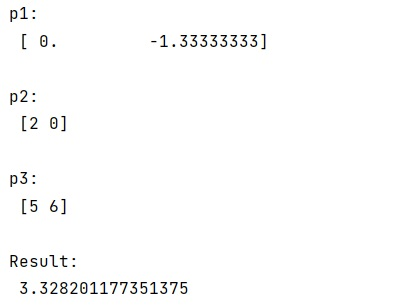# Distance between point and a line from two points in NumPy

Learn, how to find the distance between point and a line from two points in Python NumPy?
Submitted by Pranit Sharma, on February 28, 2023

NumPy is an abbreviated form of Numerical Python. It is used for different types of scientific operations in python. Numpy is a vast library in python which is used for almost every kind of scientific or mathematical operation. It is itself an array which is a collection of various methods and functions for processing the arrays.

## Finding the distance between point and a line from two points

Suppose that we are given 3 points on a coordinate plane (say p1, p2, and p3). We need to get the distance from P3 perpendicular to a line drawn between P1 and P2. We need to find a way in Python to find the distance between this point and a perpendicular line from two points.

For this purpose, we will use the numpy.cross() function. This function returns the z-coordinate of the cross-product only for 2D vectors.

We will use the norm on the difference between the two points with which we will divide the line using the cross() method.

Let us understand with the help of an example,

## Python code to find the distance between point and a line from two points in NumPy

```# Import numpy
import numpy as np

norm = np.linalg.norm

# Creating points
p1 = np.array([0,-4/3])
p2 = np.array([2, 0])
p3 = np.array([5, 6])

# Display original points
print("p1:\n",p1,"\n")
print("p2:\n",p2,"\n")
print("p3:\n",p3,"\n")

# Finding distance of p3 from a
# line connecting p1 and p2
res = norm(np.cross(p2-p1, p1-p3))/norm(p2-p1)

# Display result
print("Result:\n",res,"\n")
```

Output:Languages: » C » C++ » C++ STL » Java » Data Structure » C#.Net » Android » Kotlin » SQL
Web Technologies: » PHP » Python » JavaScript » CSS » Ajax » Node.js » Web programming/HTML
Solved programs: » C » C++ » DS » Java » C#
Aptitude que. & ans.: » C » C++ » Java » DBMS
Interview que. & ans.: » C » Embedded C » Java » SEO » HR
CS Subjects: » CS Basics » O.S. » Networks » DBMS » Embedded Systems » Cloud Computing
» Machine learning » CS Organizations » Linux » DOS
More: » Articles » Puzzles » News/Updates